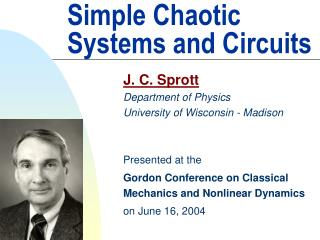# Simple Chaotic Systems and Circuits - PowerPoint PPT PresentationDownload PresentationSimple Chaotic Systems and Circuits

Download Presentation## Simple Chaotic Systems and Circuits

- - - - - - - - - - - - - - - - - - - - - - - - - - - E N D - - - - - - - - - - - - - - - - - - - - - - - - - - -
##### Presentation Transcript

1. Simple Chaotic Systems and Circuits J. C. Sprott Department of Physics University of Wisconsin - Madison Presented at the Gordon Conference on Classical Mechanics and Nonlinear Dynamics on June 16, 2004

2. Lorenz Equations (1963) dx/dt = Ay – Ax dy/dt = –xz + Bx – y dz/dt = xy – Cz 7 terms, 2 quadratic nonlinearities, 3 parameters

3. Rössler Equations (1976) dx/dt = –y – z dy/dt = x + Ay dz/dt = B + xz – Cz 7 terms, 1 quadratic nonlinearity, 3 parameters

4. Lorenz Quote (1993) “One other study left me with mixed feelings. Otto Roessler of the University of Tübingen had formulated a system of three differential equations as a model of a chemical reaction. By this time a number of systems of differential equations with chaotic solutions had been discovered, but I felt I still had the distinction of having found the simplest. Roessler changed things by coming along with an even simpler one. His record still stands.”

5. Rössler Toroidal Model (1979) “Probably the simplest strange attractor of a 3-D ODE” (1998) dx/dt = –y – z dy/dt = x dz/dt = Ay – Ay2– Bz 6 terms, 1 quadratic nonlinearity, 2 parameters

6. Sprott (1994) • 14 examples with 6 terms and 1 quadratic nonlinearity • 5 examples with 5 terms and 2 quadratic nonlinearities J. C. Sprott, Phys. Rev. E 50, R647 (1994)

7. Gottlieb (1996) What is the simplest jerk function that gives chaos? Displacement: x Velocity: = dx/dt Acceleration: = d2x/dt2 Jerk: = d3x/dt3

8. Linz (1997) • Lorenz and Rössler systems can be written in jerk form • Jerk equations for these systems are not very “simple” • Some of the systems found by Sprott have “simple” jerk forms:

9. Sprott (1997) “Simplest Dissipative Chaotic Flow” dx/dt = y dy/dt = z dz/dt = –az + y2 – x 5 terms, 1 quadratic nonlinearity, 1 parameter

10. Bifurcation Diagram

11. Return Map

12. Zhang and Heidel (1997) 3-D quadratic systems with fewer than 5 terms cannot be chaotic. They would have no adjustable parameters.

13. Linz and Sprott (1999) dx/dt = y dy/dt = z dz/dt = –az – y + |x| – 1 6 terms, 1 abs nonlinearity, 2 parameters (but one =1)

14. General Form dx/dt = y dy/dt = z dz/dt = – az – y + G(x) G(x) =±(b|x| –c) G(x) = ±b(x2/c – c) G(x) =–b max(x,0) + c G(x) =±(bx–c sgn(x)) etc….

15. Universal Chaos Approximator?

16. First Circuit

17. Bifurcation Diagram for First Circuit

18. Strange Attractor for First Circuit Calculated Measured

19. Second Circuit

20. Chaos Circuit

21. Third Circuit

22. Fourth Circuit D(x) = –min(x, 0)

23. Bifurcation Diagram for Fourth Circuit K. Kiers, D. Schmidt, and J. C. Sprott, Am. J. Phys. 72, 503 (2004)

24. http://sprott.physics.wisc.edu/ lectures/gordon04.ppt (this talk) http://www.css.tayloru.edu/~dsimons/ (circuit #4) sprott@physics.wisc.edu (to contact me) References